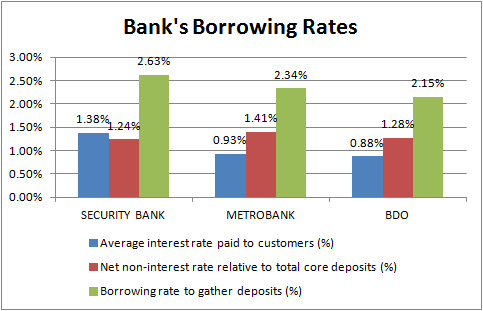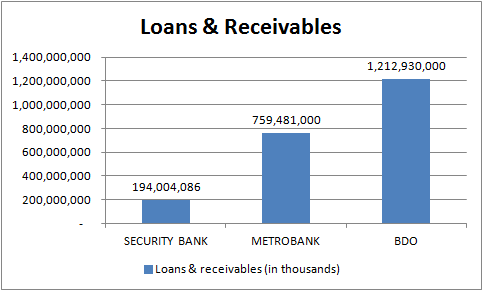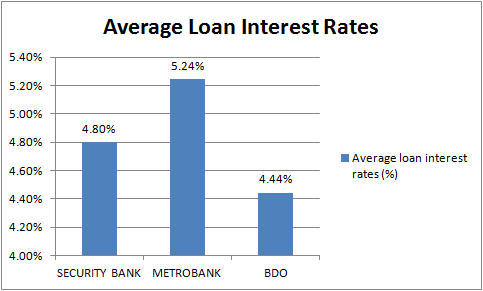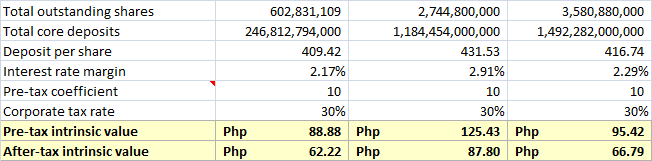Truly Rich Club Stock Picks: What Bank Stocks Should I Buy? | The Investing Engineer

# Truly Rich Club Stock Picks: What Bank Stocks Should I Buy?

Last March 10, TRC sent a stock alert instructing members to sell SMPH because it already reached the target price. For those people who sold and are enjoying their hefty gains now, congratulations. 🙂

TRC also introduced a new SAM stock, Security Bank (SECB). TRC liked the stock because the Board took in the Bank of Tokyo as a strategic partner. The Bank of Tokyo bought 20% of SECB, buying at 2.8 times its book value.

Now, I noticed that there are already three bank stock picks in the SAM table. Deciding which bank stock to choose from the list is confusing because these are all good banks.

The only question I want to answer as a value investor is which bank has the greatest value among the three.

# Truly Rich Club’s Bank Stock Picks Review

To do that, let’s try to value them and get their intrinsic value. The methods that I’ll present is based on this article which I find very useful and is the basis of how I approach bank stock valuation.

Bank’s have different financial statements when compared to other companies that’s why we need a different approach on how to value them.

What we aim at bank stock valuation is to find out the bank’s interest rate margin. Interest rate margin tells us in perspective how much money do the bank earn for every 1 peso deposited into them.

That percentage will be used to find a conservative intrinsic value based on total core deposits and outstanding shares for the particular quarter. To find this out, we need to gather data from the financial statements. (Note that the following figures presented in this post are based on the annual 2014 report.)

# Looking At The Bank’s Deposit Liabilities

One thing we need to find out in valuing banks is to know how much core deposits they get. To find it out, we must sift through the bank’s balance sheet and look for the interest-bearing and non-interest bearing deposits.

Interest-bearing deposits are entered in the balance sheet as savings, time and long-term negotiable certificate of deposits. Non-interest bearing deposits are shown as demand. The chart below shows the bank deposits for the year 2014.Fig. 1 Deposit Liabilities

Adding the two, we get the total core deposits of the three banks. It is evident that BDO has the highest total core deposits for the period covered.Fig. 2 Total Core Deposits

# Interest Expense On Deposit Liabilities: How Much Banks Pay For Their Depositors

Banks gather out deposits from us and because of that, they pay us a small percentage which we call interests. We want to know how much money they spend to pay out interests from their depositors. This data is found in the income statement called interest expense on deposit liabilities.Fig. 3 Interest Expense On Deposit Liabilities

The data shows that the more core deposits the bank has, the higher the interest expense on deposit liabilities it also has.

With these values, we can now get the average interest rate paid out to depositors. I’ll explain that later as we go on.

# Net Non-interest Expense

Net non-interest expense tells us how much money the bank spends independent of interest expense. To get that value, we subtract the total operating income (non-interest income) to the total operating expense (non-interest expense). These values are found in the income statement.Fig. 4 Net Non-Interest Expense

With these values, we can get the percentage of net non-interest expense relative to total core deposits which will be used to get the bank’s borrowing rate to gather deposits.

# The Average Borrowing Rate

The bank’s average borrowing rate to gather deposits give us an overview on how much money the banks pay out to depositors for every 1 peso they get.

To get the borrowing rate, we need to add the average interest rate paid to customers and the percentage of net non-interest expense relative to total core deposits.

• To get the average interest rate paid to customers, we need to divide the interest expense on deposit liabilities to the total interest-bearing deposit liabilities.
• To get the percentage of net non-interest expense relative to total core deposits, we just need to divide former to the latter.

The data below shows the borrowing rate of the three bank stocks.Fig. 5 Bank’s Borrowing Rates

In perspective, for every 1.00 Peso deposit;

• SECB pays out 0.03 Pesos.
• MBT pays out 0.02 Pesos.
• BDO pays out 0.02 Pesos.

This also tells us that SECB is paying more interests to its depositors despite of lower core deposits compared to the two.

# Average Loan Interest Rate

The average loan interest rate is the interest rate they impose when they loan out the money to borrowers. To get this percentage rate, we divide the interest income on loans to loans and receivables assets.

These can be found on the income statement and the balance sheet respectively.Fig. 6 Interest Income On LoansFig. 7 Loans & Receivables

With the data given above, we can now compute for the average loan rate.Fig. 8 Average Loan Interest Rates

In perspective, it tells us that for every 1 Peso they loan out to borrowers;

• SECB earns 0.05 Pesos.
• MBT earns 0.05 Pesos.
• BDO earns 0.04 Pesos

In this data, it tells us that MBT earns more on loans compared to the other two banks.

# Interest Rate Margin; The Measure Of How Profitable A Bank Is

The interest rate margin measures how much money the bank earns for every 1 peso deposited into them. It is computed by subtracting the average borrowing rate to the average loan interest rates.

By looking at the value above and applying the formula, we get the following interest rate margins;Fig. 9 Interest Rate Margin

What this data tells us is that for every 1.00 Peso deposited;

• SECB earns 0.02 Pesos.
• MBT earns 0.03 Pesos.
• BDO earns 0.02 Pesos.

We like banks with higher interest rate margins because it means higher earnings on the money deposited into them. By taking in our money as a liability by paying us interest, they lend it out at higher rates to offset their interest expense. This is how a bank makes money. The three banks mentioned almost make 3% on our deposits, MBT being the highest making 2.91% off their customer’s deposits.

# Calculating Intrinsic Value Based On Deposits

We need to calculate the deposit per share. It is calculated by dividing the total core deposits to the total outstanding shares for that period. We will also assign a pre-tax coefficient equal to 10. You may also use the bank’s industry standard P/E ratio as a substitute to the pre-tax coefficient if you wish.

To calculate the pre-tax intrinsic value, we multiply the deposit per share, pre-tax coefficient and the interest rate margin.

To calculate the after-tax intrinsic value, we will need to get the corporate tax rate which is 30% here in the Philippines and multiply it to the pre-tax intrinsic value. The calculated value is then subtracted to the pre-tax intrinsic value.

Here’s the summary of the equations described above.Fig. 10 From left to righ: SECB, MBT, BDO

# What Does This Mean?

Here are the pre-tax intrinsic values;

• SECB – Php 88.88
• MBT – Php 125.43
• BDO – Php 95.42

When deciding to buy a bank stock, we should consider buying at a price lower than the calculated intrinsic value. Looking at the closing prices as of today, March 16, 2106;

• SECB – Php 155.80
• MBT – Php 84.35
• BDO – Php 104.00

It seems that only MBT trades lower than the intrinsic value. So based on this intrinsic value calculation, we conclude that MBT is the better bank stock to buy among the three.

# Final Thoughts

You should also consider broker fair value estimates for comparison. COL Financial and Truly Rich Club’s FV estimate is at Php 92.00 so technically it’s a ‘buy’ even though TRC mentions it as ‘stop buying’ as of today.

Remember that this is a conservative approach and only used deposits as the basis of the calculation. Banks also make profit on other things so you need to consider that as well. But always remember the main value lies on the deposits they get and how much money they make from it.

The Truly Rich Club stock picks are listed in the SAM Table. You can follow the investing guide if you join here.

Happy investing!

### **BONUS** Click here to download Stocks Updates and Wealth Strategies sample PDF reports for FREE!

#### Model Portfolios: Unloved, Value Play, Yield Seeker, Riding the Momentum (15 May 2019)

Looking for model portfolios to guide your stock picking decisions? Don't worry, we got you covered! Check out this special stock picks and model portfolio report today! (Needs premium access.)

•Zoren Musngi says:

Hi. I appreciate the detail and effort you have put into trying to value the banks. Valuing banks are indeed tricky. However, i do not understand why you used only deposits in calculating an intrinsic value. Remember that a company, in order to start a business, has two sources of capital, equity and debt. For a bank, another type is added into the equation; deposits, which are considered liabilities. So a bank’s sources of capital are from: equity (stockholders), debt (creditors / bonds), deposits (depositors).

These three items all equal to the bank’s total assets, which comprise of Loans, Investment Securities, Cash on Hand, Receivables, Equipment, etc. So if you want to value banks based on its holdings or asset (kinda like NAV), i think you need to take a holistic view.

In valuing banks, i suggest using relative Price to Book (P/B) analysis and Excess Return Models (See Aswath Damodaran’s paper here: http://people.stern.nyu.edu/adamodar/pdfiles/papers/finfirm09.pdf).

Now, in assessing the operations, i suggest looking at the following metrics:
1. Return on Equity
2. Return on Assets
3. Leverage Ratio
4. Capital Ratios
5. Cost-to-Income ratio
6. Net Interest Margin (its already disclosed by banks in their press releases and information statements)
7. Non-Performing Loans (NPL) as % of Loans
8. Earnings mix between interest income and fee income
9. Loan concentration / exposure (commercial, consumer loans, other)

Let me know if you have concerns. I wish you good luck on your journey in the capital markets. 🙂

•The Investing Engineer PH says:

Hi Zoren,

Thank you for the wonderful insight. 🙂

Yes the calculations are just based on deposits. It doesn’t include other assets that a bank may have. This approach is very conservative that’s why I also made a comparison on a broker’s FV estimates.

Thank you for the info you provided as this will definitely help me to better my valuation knowledge. 🙂

Godbless.